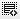## question - 3 Regarding Series execution0

s1 = pd.Series([1,2,3,4,5])
s2 = pd.Series(['A','B','C','D','E'])
s3 = pd.Series(['Q','W','E','R','T','U'])

pd.DataFrame(
[s1,s2,s3],
#index = ["Row 1","Row 2","Row 3"],
#columns = ["First","Second","Third","Fourth","Fifth","Sixth"],
)

output

0    1    2    3    4    5
0    1    2    3    4    5    NaN
1    A    B    C    D    E    NaN
2    Q    W    E    R    T    U

but

when

s1 = pd.Series([1,2,3,4,5])
s2 = pd.Series(['A','B','C','D','E'])
s3 = pd.Series(['Q','W','E','R','T','U'])

pd.DataFrame(
[s1,s2,s3],
index = ["Row 1","Row 2","Row 3"],
columns = ["First","Second","Third","Fourth","Fifth","Sixth"],
)
output

First    Second    Third    Fourth    Fifth    Sixth
Row 1    NaN    NaN    NaN    NaN    NaN    NaN
Row 2    NaN    NaN    NaN    NaN    NaN    NaN
Row 3    NaN    NaN    NaN    NaN    NaN    NaN

problem is all is set to NaN why ?

Click on thisicon to add code snippet.